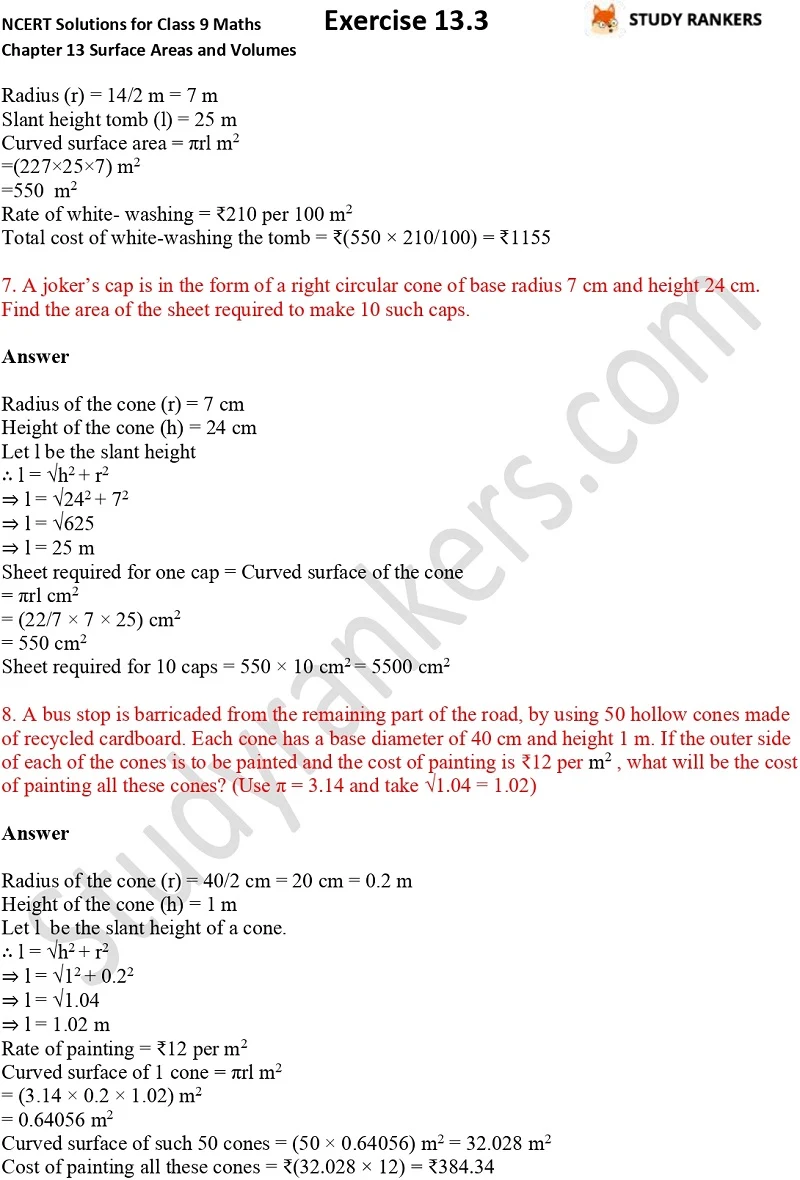## NCERT Solutions for Class 9 Maths Chapter 13 Surface Areas and Volumes Exercise 13.3

If you want Chapter 13 Surface Areas and Volumes Exercise 13.3 Class 9 Maths NCERT Solutions then you can find them here which will help you in understanding the basics of the chapter. NCERT Solutions for Class 9 Maths will make you aware about the concepts and important formulas which will be helpful a lot in solving questions. These NCERT questions and answers are updated as per the latest syllabus of CBSE. The answers provided here are detailed so you can understand every concepts given in the question easily and also practice yourself and check later.

There are total 8 questions in the chapter which are finding total surface area and curved surface area of given solid.